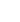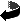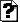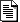# Index of /code/glm-ie/pls/lbfgsbNameLast modifiedSizeDescriptionParent Directory  -Makefile2019-03-23 09:59 2.2KREADME.html2019-03-23 09:59 18Karray.h2019-03-23 09:59 4.4Karrayofmatrices.cpp2019-03-23 09:59 2.4Karrayofmatrices.h2019-03-23 09:59 2.1Klbfgsb.cpp2019-03-23 09:59 4.0Klicense.txt2019-03-23 09:59 1.5Kmatlabexception.cpp2019-03-23 09:59 512matlabexception.h2019-03-23 09:59 869matlabmatrix.cpp2019-03-23 09:59 1.6Kmatlabmatrix.h2019-03-23 09:59 2.4Kmatlabprogram.cpp2019-03-23 09:59 7.3Kmatlabprogram.h2019-03-23 09:59 2.8Kmatlabscalar.cpp2019-03-23 09:59 1.0Kmatlabscalar.h2019-03-23 09:59 1.2Kmatlabstring.cpp2019-03-23 09:59 1.0Kmatlabstring.h2019-03-23 09:59 1.1Kprogram.cpp2019-03-23 09:59 3.9Kprogram.h2019-03-23 09:59 4.9Ksolver.f2019-03-23 09:59 138K

MATLAB interface for L-BFGS-B

## A MATLAB interface for L-BFGS-B

by Peter Carbonetto
Dept. of Computer Science
University of British Columbia

L-BFGS-B is a collection of Fortran 77 routines for solving large-scale nonlinear optimization problems with bound constraints on the variables. One of the key features of the nonlinear solver is that knowledge of the Hessian is not required; the solver computes search directions by keeping track of a quadratic model of the objective function with a limited-memory BFGS (Broyden-Fletcher-Goldfarb-Shanno) approximation to the Hessian.1 The algorithm was developed by Ciyou Zhu, Richard Byrd and Jorge Nocedal. For more information, go to the original distribution site for the L-BFGS-B software package.

I've designed an interface to the L-BFGS-B solver so that it can be called like any other function in MATLAB.2 See the text below for more information on installing and calling it in MATLAB. Along the way, I've also developed a C++ class that encapsulates all the "messy" details in executing the L-BFGS-B code. See below for instructions on how to use this class for your own C++ code.

If you have any questions, praise, or comments, or would like to report a bug, do not hesitate to contact the author. I've tested this software in MATLAB version 7.2 on both the Mac OS X and Linux operating systems.This work is licensed under a Creative Commons Attribution-Noncommercial-Share Alike 2.5 Canada License. Please cite the source as Peter Carbonetto, Department of Computer Science, University of British Columbia.

#### Installation

Download the source. First, download and unpack the tar archive. It contains some MATLAB files (ending in .m), some C++ source and header files (ending in .h and .cpp), a single Fortran 77 source file solver.f containing the L-BFGS-B routines, and a Makefile. I've included a version of the Fortran routines that is more recent than what is available for download at the distribution site at Northwestern University.

What we will do is create a MEX file, which is basically a file that contains a routine that can be called from MATLAB as if it were a built-it function. To learn about MEX files, I refer you to this document at the MathWorks website.

Install the C++ and Fortran 77 compilers. In order to build the MEX file, you will need both a C++ compiler and a Fortran 77 compiler. Unfortunately, you can't just use any compiler. You have to use the precise one supported by MATLAB. For instance, if you are running Mac OS X 7.2 on a Linux operating system, you will need to install the GNU compiler collection (GCC) version 3.4.4. Even if you already have a compiler installed on Linux, it may be the wrong version, and if you use the wrong version things could go horribly wrong! It is important that you use the correct version of the compiler, otherwise you will encounter linking errors. Refer to this document to find out which compiler is supported by your version of MATLAB.

Configure MATLAB. Next, you need to set up and configure MATLAB to build MEX Files. This is explained quite nicely in this MathWorks support document.

Modify the Makefile. You are almost ready to build the MEX file. But before you do so, you need to edit the Makefile to coincide with your system setup. At the top of the file are several variables you may need to modify. The variable `MEX` is the executable called to build the MEX file. The variables `CXX` and `F77` must be the names of your C++ and Fortran 77 compilers. `MEXSUFFIX` is the MEX file extension specific to your platform. (For instance, the extension for Linux is `mexglx`.) The variable `MATLAB_HOME` must be the base directory of your MATLAB installation. Finally, `CFLAGS` and `FFLAGS` are options passed to the C++ and Fortran compilers, respectively. Often these flags coincide with your MEX options file (see here for more information on the options file). But often the settings in the MEX option file are incorrect, and so the options must be set manually. MATLAB requires some special compilation flags for various reasons, one being that it requires position-independent code. These instructions are vague (I apologize), and this step may require a bit of trial and error until before you get it right.

On my laptop running Mac OS X 10.3.9 with MATLAB 7.2, my settings for the Makefile are

``````  MEX         = mex
MEXSUFFIX   = mexmac
MATLAB_HOME = /Applications/MATLAB72
CXX         = g++
F77         = g77
CFLAGS      = -O3 -fPIC -fno-common -fexceptions \
-no-cpp-precomp
FFLAGS      = -O3 -x f77-cpp-input -fPIC -fno-common``````

On my Linux machine, I set the variables in the Makefile like so:

``````  MEX         = mex
MEXSUFFIX   = mexglx
MATLAB_HOME = /cs/local/generic/lib/pkg/matlab-7.2
CXX         = g++-3.4.5
F77         = g77-3.4.5
FFLAGS      = -O3 -fPIC -fexceptions``````

It may be helpful to look at the GCC documentation in order to understand what these various compiler flags mean.

Build the MEX file. If you are in the directory containing all the source files, typing `make` in the command prompt will first compile the Fortran and C++ source files into object code (.o files). After that, the make program calls the MEX script, which in turn links all the object files together into a single MEX file. If you didn't get any errors, then you are ready to try out the bound-constrained solver in MATLAB. Note that even if you didn't get any errors, there's still a possibility that you didn't link the MEX file properly, in which case executing the MEX file will cause MATLAB to crash. But there's only one way to find out: the hard way.

#### A brief tutorial

I've written a short script `examplehs038.m` which demonstrates how to call `lbfgsb` for solving a small optimization problem, the Hock & Schittkowski test problem no. 38.3 The optimization problem has four variables. They are bounded from below by -10 and bounded from above by +10. We've set the starting point to (-3, -1, -3, -1). We've written two MATLAB functions for computing the objective function and the gradient of the objective function at a given point. These functions can be found in the files `computeObjectiveHS038.m` and `computeGradientHS038.m`. If you run the script, the solver should very quickly progress toward the point (1, 1, 1, 1). The objective is 0 at this point.

The basic MATLAB function call is

``  lbfgsb(x0,lb,ub,objfunc,gradfunc)``

The first input argument `x0` declares the starting point for the solver. The second and third input arguments define the lower and upper bounds on the variables, respectively. If an entry of `lb` is set to `-Inf`, then this is equivalent to no lower bound for that entry (the same applies to upper bounds, except that it must be `+Inf` instead). The next two input arguments must be the names of MATLAB routines (M-files). The first routine must calculate the objective function at the current point. The output must be a scalar representing the objective evaluated at the current point. The second function must compute the gradient of the objective at the current point. The input is the same as `objfunc`, but it must return as many outputs as there are inputs. For a complete guide on using the MATLAB interface, type `help lbfgsb` in the MATLAB prompt.

The script `exampleldaimages.m` is a more complicated example. It uses the L-BFGS-B solver to compute some statistics of a posterior distribution for a text analysis model called latent Dirichlet allication (LDA). The model is used to estimate the topics for a collection of documents. The optimization problem is to compute an approximation to the posterior, since it is not possible to compute the posterior exactly. In this case, the optimization problem corresponds to minimizing the distance between the true posterior distribution and an approximate distribution. For more information, see the paper of Blei, Ng and Jordan (2003).4 In order to run this example, you will need to install the lightspeed toolbox for MATLAB developed by Tom Minka at Microsoft Research.

The script starts by generating a bunch of canonical images that represent topics; if a pixel is white then a word at that position is more likely to appear in that topic. Note that the order of the pixels in the image is unimportant, since LDA is a "bag of words" model. In another figure we show a small sample of the data generated from the topics. Each image represents a document, and the pixels in the image depict the word proportions in that document.5

Next, the script runs the L-BFGS-B solver to find a local minimum to the variational objective. Buried in the M-file `mflda.m` is the call to the solver:

``````  [xi gamma Phi] = lbfgsb({xi gamma Phi},...
{repmat(lb,W,K)  repmat(lb,K,D)  repmat(lb,K,M)},...
{repmat(inf,W,K) repmat(inf,K,D) repmat(inf,K,M)},...
{nu eta L w lnZconst},'callbackMFLDA',...
'm',m_lbfgs,'factr',1e-12,'pgtol',...
tolerance,'maxiter',maxiter);``````

This example will give us the opportunity to demonstrate some of the more complicated aspects of the our MATLAB interface. The first input to `lbfgsb` sets the initial iterate of the optimization algorithm. Notice that we've passed a cell array with entries that are matrices. Indeed, it is possible to pass either a matrix or a cell array. Whatever structure is passed, the other input arguments (and outputs) must also abide by this structure. The lower and upper bounds on variables are also cell arrays. All the upper bounds are set to infinity, which means that the variables are only bounded from below.

It is instructive to examine the callback routines. They look like:

``````  f = computeObjectiveMFLDA(xi,gamma,Phi,auxdata)

[dxi, dgamma, dPhi] = ...

Notice that each entry to the cell array is its own input argument to the callback routines. The same applies for the output arguments of the gradient callback function.

The sixth input argument is a cell array that contains auxiliary data. This data will be passed to the MATLAB functions that evaluate the objective and gradient. The seventh input argument specifies a callback routine that is called exactly once for every iteration. This callback routine is most useful for examining the progress of the solver. The remaining input arguments are label/value pairs that override some of the default settings of L-BFGS-B.

After converging to a solution, we display topic samples drawn from the variational approximation. A good solution should come close to recovering the true topics from which the data was generated (although they might not be in the same order).

Don't be surprised if it takes many iterations before the optimization algorithm converges on a local minimum. (In repeated trials, I found it as many as two thousand iterations to converge to a stationary point of the objective.) This particular problem demonstrates the limitations of limited-memory quasi-Newton approximations to the Hessian; the low storage requirements can come at the cost of slow convergence to the solution.

#### The C++ interface

One of the nice byproducts of writing a MATLAB interface for L-BFGS-B is that I've ended up with a neat little C++ class that encapsulates the nuts and bolts of executing the solver. A brief description of the Program class can be found in the header file `program.h`.

Program is an abstract class, which means that its impossible to instantiate a Program object. This is because the class has member functions that aren't yet implemented. These are called pure virtual functions. In order to use the Program class, one needs to define another class that inherits the Program class and that implements the pure virtual functions. In fact, the class MatlabProgram (in `matlabprogram.h`) is an example of such a class.

The new child class must declare and implement these two functions:

``````  virtual double computeObjective (int n, double* x);
virtual void computeGradient (int n, double* x, double* g); ``````

See the header file for a detailed description of these functions. The only remaining detail is calling the Program constructor. After that, it is just a matter of declaring a new object of type MyProgram then calling the function runSolver.

#### Contents of the tar archive

`solver.f`
Fortran 77 source for the L-BFGS-B solver routines.

`program.hprogram.cpp`
Header and source file for the C++ interface.

```array.h arrayofmatrices.h matlabexception.h matlabmatrix.h matlabprogram.h matlabscalar.h matlabstring.h arrayofmatrices.cpp matlabexception.cpp matlabmatrix.cpp matlabprogram.cpp matlabscalar.cpp matlabstring.cpp lbfgsb.cpp ```
Header and source files for the MATLAB interface.

`lbfgsb.m`
Usage instructions for the MEX file.

`examplehs038.m`
MATLAB script for solving the H&S test example no. 38.

```computeObjectiveHS038.m computeGradientHS038.m genericcallback.m ```
MATLAB functions used by the script `examplehs038.m`.

`exampleldaimages.m`
MATLAB script that generates synthetic documents and topics, then computes a mean field variational approximation to the posterior distribution of the latent Dirichlet allocation model, then displays the result.

```callbackMFLDA.m computeObjectiveMFLDA.m computeGradientMFLDA.m computelnZ.m computelnZconst.m computem.m dirichletrnd.m gammarnd.m createsyntheticdata.m mfldainit.m ```
Some functions used by `exampleldaimages.m` and `mflda.m`.

#### Footnotes

1 Ciyou Zhu, Richard H. Byrd, Peihuang Lu and Jorge Nocedal (1997). Algorithm 778: L-BFGS-B: Fortran subroutines for large-scale bound-constrained optimization. ACM Transactions on Mathematical Software, Vol. 23, No. 4., pp. 550-560.

1 Another MATLAB interface for the L-BFGS-B routines has been developed by Liam Stewart at the University of Toronto.

3 Willi Hock and Klaus Schittkowski (1981). Test Examples for Nonlinear Programming Codes. Lecture Notes in Economics and Mathematical Systems, Vol. 187, Springer-Verlag.

4 David M. Blei, Andrew Y. Ng, Michael I. Jordan (2003). Latent Dirichlet allocation. Journal of Machine Learning Research, Vol. 3, pp. 993-1022.

5 Tom L. Griffiths and Mark Steyvers (2004). Finding scientific topics. Proceedings of the National Academy of Sciences, Vol. 101, pp. 5228-5235.

March 3, 2007